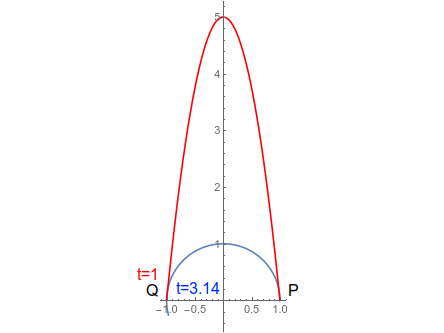# Two people walk from P (1, 0) to the point Q (-1, 0) along the paths r_1 (t) = < cos t, sin t >...

## Question:

Two people walk from {eq}P (1,\ 0) {/eq} to the point {eq}Q (-1,\ 0) {/eq} along the paths {eq}r_1 (t) = \langle \cos t,\ \sin t \rangle {/eq} and {eq}r_2 (t) = \langle -t,\ -5 (t^2 - 1) \rangle {/eq} respectively. Who arrives at {eq}Q {/eq} first and why? Graph each curve and indicate the time when they reach point {eq}Q {/eq}.

## Time Dependent Position

The coordinates of the position of a particle can be expressed as function of the time parameter. With that, it can be found the time at which the particle reaches to a given point. Time is not necessarily constrained to the positive values. Negative values means time before time parameter starts to be measured.

We must find which times correspond to each point for each path. In the first path, we have:

{eq}\begin{align} r_1(t)=\left(\cos t,\sin t\right)\\ P(1,0)=\left(\cos t,\sin t\right)\\ t_{1,P}=0\\ Q(-1,0)=\left(\cos t,\sin t\right)\\ t_{1,Q}=\pi\\ \end{align} {/eq}

and for the second path:

{eq}\begin{align} r_2(t)=\left(-t,-5(t^2-1)\right)\\ P(1,0)=\left(-t,-5(t^2-1)\right)\\ t_{1,P}=-1\\ P(-1,0)=\left(-t,-5(t^2-1)\right)\\ t_{1,Q}=1\\ \end{align} {/eq}

Then the person moving along the second path arrives first at point Q (at t=1).